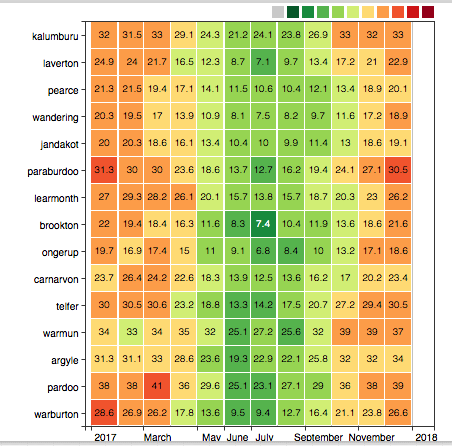# Calendar Chart

# Overview

The Calendar Chart displays the deviation of aggregated series values for a calendar period from a specified threshold. Series values within each period are aggregated by client statistical function and assigned a color which reflects the magnitude of deviation.

[widget]
type = calendar

thresholds = 0,30,60,100
colors = green,yellow,red

timespan = 12 hour
summarize-period = 1 hour
summarize-statistic = percentile(95)

[series]
metric = cpu_busy
entity = nurswgvml0*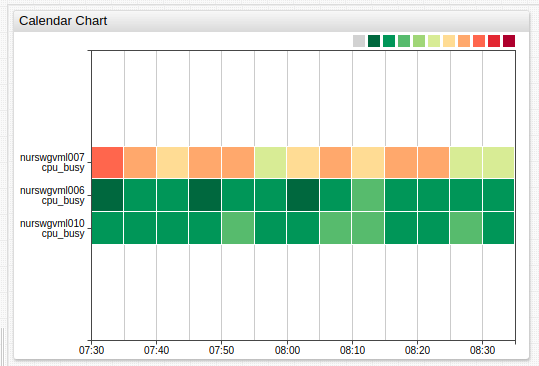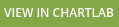# Widget Settings

• The settings apply to the [widget] section.
• Common [widget] settings are inherited.
Name Description
summarize-period Period specified in time units to split loaded time series into calendar segments.
Format: count time_unit.
Default value: 5 minute.
Example: summarize-period = 1 hour
summarize-statistic Statistical function applied to values within each period.
Refer to Client Aggregators for possible values.
Default value: avg.
Example: summarize-statistic = percentile(75)
palette-ticks Display legend labels.
Possible values: false, true.
Default Value: false.
Example: palette-ticks = true
rotate-palette-ticks Rotate legend labels.
Possible values: false (horizontal), true (vertical).
Default value: false.
Example: rotate-palette-ticks = true
range-merge Compute a single range for all series with minimum and maximum from all loaded series.
Possible values: false, true.
Default value: false.
Example: range-merge = true
sort Sort rows by label or value of statistic function. Supported ascending (ASC) and descending (DESC) order.
Possible values:
name - sorts by label
stat_name('count unit')- sorts by statistic within period
Example: sort = name DESC

centralize-ticks Center time and date markers over summarize periods.
Possible values: false, true.
Default value: false.
Example: centralize-ticks = true
ticks-time Tick marks on the time axis.
Default value: 10.
Example: ticks-time = 5
data-labels Display values inside colored rectangles formatted according to format setting.
Default value: false.
Example: data-labels = true

# Summarize Period Panel

Name Description
display-panels Summarize-period panel visibility.
Possible values: false, true, hover.
Default value: hover.
Example: display-panels = true
expand-panels Expand controls in the summarize-period panel.
Possible values: false, true, compact.
Default value: compact.
Example: expand-panels = true
periods List of custom aggregation periods on the summarize-period panel.
Each period is specified as the number of time units.
Format: count time_unit.
Example: periods = 20 minute, 4 hour. The periods must be comma-separated.
panels-period Comma-separated list of aggregation periods to replace the default ones in summarize-period panel.
Example: panels-period = 1 hour, 1 day, 1 week

Panel features:

• Double-click between controls to insert an inner interval: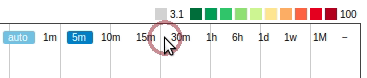• Drag to drop control: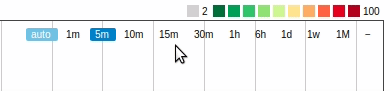# Series Color Settings

• The settings apply to the [widget] or [series] section.
• Common [series] settings are inherited.
Name Description
thresholds Threshold values.
The number of specified colors must be 1 fewer than the number of thresholds.
Example: thresholds = 0, 50, 90, 100
colors Comma separated list of color names or hex codes, assigned to threshold ranges.
The number must be 1 fewer than the number of thresholds.
Example: colors = green, yellow, red
Recommended color palette tools: colorhexa, material.io.
color-range Color palette assigned to threshold ranges.
Possible values: color names.
Built-in ranges: red, blue, black.
Example: color-range = black
Default value: 3.

# Sort By Statistic

Aggregated series can be sorted using statistic, calculated for last aggregated values within the particular period, specified as the number of time units:

stat_name('count unit')
# For example:
sum('5 minute')

Period can be both quoted and unquoted. Supported statistic functions:

• sum - sum of values during the period
• min - minimum value during the period
• max - maximum value during the period
• avg - average of values during the period
• first - first value received during the period
• last - Last value received during the period# Examples

# Data Labels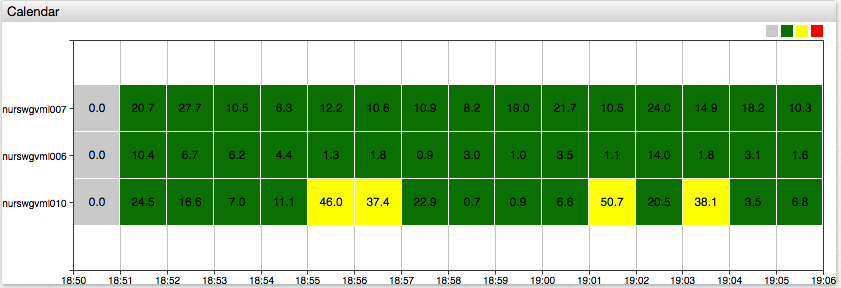# Custom Color Range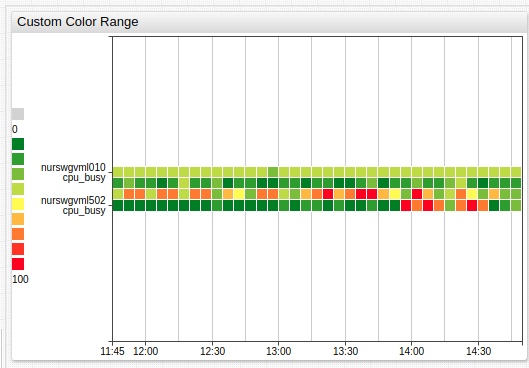# Legend Position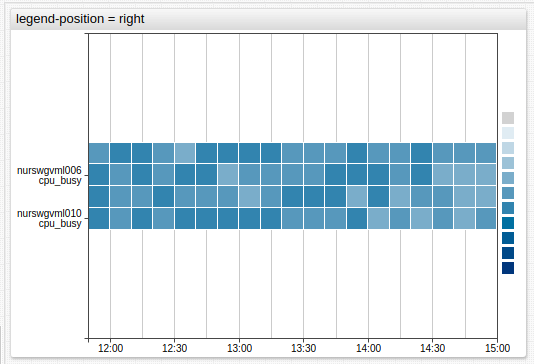# No Threshold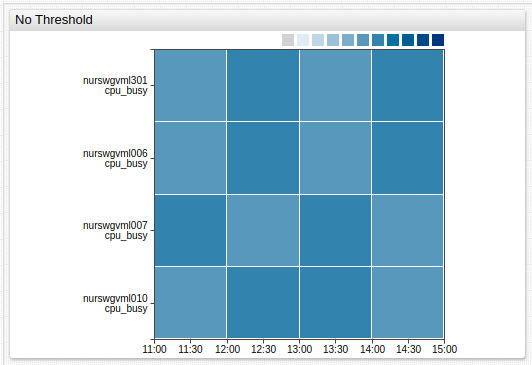# Threshold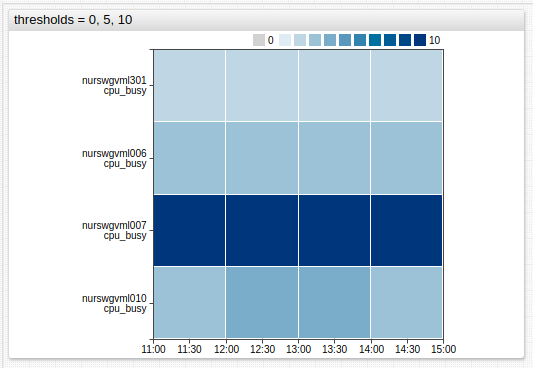# Multiple Weather Stations